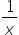Maths-
General
Easy

###Hint:

We are given a function. We have to find if it's decreasing or increasing. A function is said to be decreasing if it's first order derivative is negative. A function is increasing if it's first order derivative is positive. So, we will take the first order derivative of the given function.

#### The given function isf(x) = 1 +We will find the first order derivative of the given function.The quantity x2  will be always positive.So, the value of the function due to the negative sign will be always negativeIt means f'(x) < 0So, the given function is always decreasing.

We have to see the rules for a increasing and decreasing function.

## Book A Free Demo+91